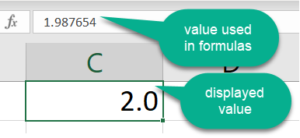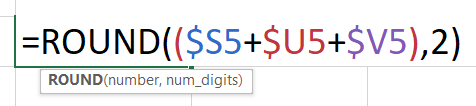# Excel ROUND Function: Flaw or Hidden Truths?

## The Problem with Excel Formatting

I occasionally receive email messages from clients and contacts about a Microsoft Excel “problem”, that is, each one asked a similar question, “Is there a bug in Excel? It looks like some of my formulas are wrong because I get a different answer when I manually calculate the same values.”

No, this isn’t a bug. Instead, it’s a rounding error. Have you heard of the acronym WYSIWYG (pronounced whizzy-wig)? That is, What You See Is What You Get. In some Excel worksheets, however, WYSIWYG means What You See ISN’T What You Get. The frustration begins when you cannot duplicate the Excel answers when you manually calculate visible values.

## See It for Yourself

To try a simple example of how Excel formatting can change a displayed value but not the “real” value:1. Open Microsoft Excel (any version).
2. In a new worksheet, in any cell, type this number: 1.987654
3. Select the cell and click repeatedly on the Decrease Decimal toolbar/icon found on the Home Tab/Number Group, and watch the changes to the display until the value shows as 2.0.
4. Look in the formula bar to verify the original value hasn’t changed; this value is what Excel uses in formulas. The displayed number of 2.0 is what your readers will likely use if they audit your work.

TIP #1: When you select a popular number formatting option in Excel, like currency or comma, Excel will round the displayed results to the specified number of decimal places, such as 2 or 0. The obstacle is that these formatting actions do not change or round the value behind the result to the same number of decimal places. Instead, Excel retains the full value as it is passed on to other formulas in the worksheet.

## Disclaimers Aren’t the Solution

Have you seen or used disclaimers in worksheets that state “errors may occur due to rounding?” With statements like these, how can the reviewers of your Excel data trust the accuracy and reliability of the results and the decisions based on this information?

You may not have encountered this problem if the numbers you use in Excel are only whole numbers or limited to dollars and cents (substitute your country’s currencies). Many accounting and financial applications, however, include percentages, fractional shares, and other values that may calculate out to many more decimal places than you choose to display. These calculation problems are common, for instance, in oil and gas and other industries where volumes, lease ownerships, prices, and other factors will often be quantified out to 3, 6, 12, or more decimal places.

To give you an idea of how critical this issue can be, I was hired by an oil analyst for an “emergency consultation” when a shareholder threatened to sue the company because the numbers on his property expense worksheet literally “didn’t add up.”

## What’s the Answer? The Excel ROUND Function

To change your Excel formulas so that the displayed answers match the values behind the results, add the ROUND function to your formulas. Using the ROUND function, you can calculate your values using the same number of decimal places that you want to display in your worksheet.

The basic structure for the ROUND function is:=ROUND(formula, # of decimal places)

The official Excel lingo is =ROUND(number, num_digits)

The ROUND function can be easily applied to even very complex formulas. Just add it as the outermost function to any formula, such as =ROUND(S5*(H5+J5),2).

With some Excel functions and calculations, the ROUND function may also be nested within other functions. Here is an example of the Excel ROUND function included in the arguments (parts) of the IF function. This ensures the result is calculated to the correct number of decimal places.

=IF((W5*100)>0,ROUND((W5*100),0),ROUND((-W5*100),0))

TIP #2: The number of decimal places in the ROUND function should always match the number of decimal places chosen for the formatting of the cell. This way, you can actually create worksheets and formulas that are WYSIWYG.

TIP #3: Even though some formulas may create correct results without the ROUND function, be consistent so you can automatically rule out rounding problems when auditing worksheets.

To extend your learning on Microsoft Excel, check out these additional shortcuts, tips, and techniques at TheSoftwarePro.com/Excel.

© Dawn Bjork, MCT, MOSM, CSP®, The Software Pro®
Microsoft Certified Trainer, Productivity Speaker, Certified Speaking Professional

#### Related Posts